person
Computer Science Arts English Psychology Yoga Entrepreneurship
but you can still pick the better path.
AstraPath is a growing collection of free resources you can use to learn a new subject or skill, ranging from video lectures to text-based tutorials.
by coco Drawabox by guest by Starswheat by Starswheat IB Guides by astra Problem Set 1 Solutions by elizabethmcclaire by 270392840
and learn from others.
AstraNote not just your notepad, but also a web of notes. Simply put, you get to use notes from other learners, and others can also see yours.
Of course, you also have the option to keep all your notes private.

123

## 2.1.1 Define displacement, velocity, speed and acceleration.

Displacement
Displacement is the distance moved in a particular direction. It is a vector quantity.

SI unit: m
Symbol: s

Velocity
Velocity is the rate of change of displacement. It is a vector quantity.
Velocity = (change in displacement / change in time)

SI unit: m s-1
Symbol: v or u

Speed
Speed is the rate of change of distance. It is a scalar quantity.
Speed = (change in distance / change in time)

SI unit: m s-1
Symbol: v or u

Note that speed and velocity are not the same thing. Velocity has a direction.

Acceleration
Acceleration is the rate of change of velocity. It is a vector quantity.
Acceleration = (change in velocity / change in time)

SI unit: m s-2
Symbol: a

Note that acceleration is any change in velocity, meaning an increase or decrease in velocity or a change in direction.

## 2.1.2 Explain the difference between instantaneous and average values of speed, velocity and acceleration.

Instantaneous
An instantaneous value of speed, velocity or acceleration is one that is at a particular point in time.

Average
An average value of speed, velocity or acceleration is one that is taken over a period of time.

## 2.1.3 Outline the conditions under which the equations for uniformly accelerated motion may be applied.

The equations of uniformly accelerated motion can only be under conditions where the acceleration is constant.

The equations of uniformly accelerated motion are as follows:

 Variable Symbol t time taken s distance travelled u initial velocity v final velocity a acceleration

Table 1.2.1 - Variables used in uniformly accelerated motion equations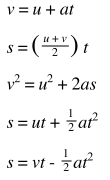Other equations may be derived from these equations.

## 2.1.4 Identify the acceleration of a body falling in a vacuum near the Earth?s surface with the acceleration g of free fall.

When we ignore the effect of air resistance on an object falling down to earth due to gravity we say the object is in free fall. Free fall is an example of uniformly accelerated motion as the only force acting on the object is that of gravity.

On the earths surface, the acceleration of an object in free fall is about 9.81 ms-1. We can easily recognise the uniform acceleration in displacement - time, velocity - time and acceleration - time graphs as shown below:

## 2.1.5 Solve problems involving the equations of uniformly accelerated motion.

A car accelerates with uniformly from rest. After 10s it has travelled 200 m.

Calculate:

Its average acceleration

S = ut + 1/2 at²

200 = 0 x 10 +  1/2  x a x 10²

200 = 50a

a = 4 m s-2

Its instantaneous speed after 10s

v² = u ² + 2as

= 0 + 2 x 4 x 10

= 80

V= 8.9 m s-1

## 2.1.6 Describe the effects of air resistance on falling objects.

Air resistance eventually affects all objects that are in motion. Due to the effect of air resistance objects can reach terminal velocity. This is a point by which the velocity remains constant and acceleration is zero.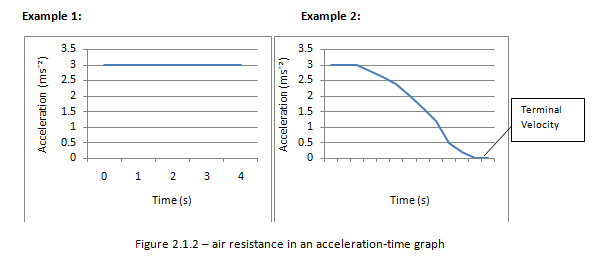In the absence of air resistance all objects have the same acceleration irrespective of its mass.

## 2.1.7 Draw and analyse distance?time graphs, displacement?time graphs, velocity?time graphs and acceleration?time graphs.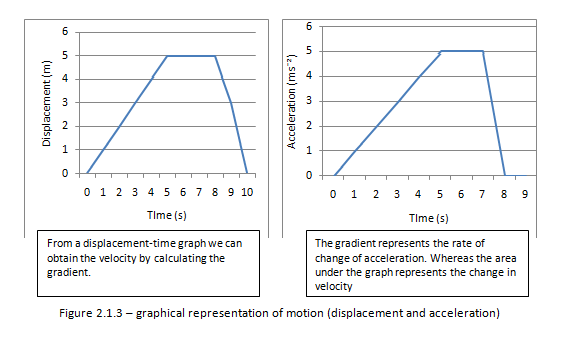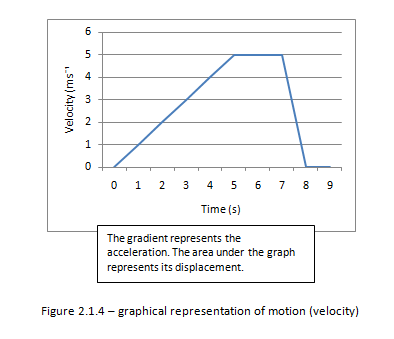## 2.1.8 Calculate and interpret the gradients of displacement?time graphs and velocity?time graphs, and the areas under velocity?time graphs and acceleration?time graphs.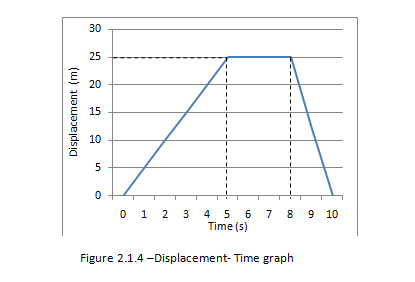Determining its velocity
We know that the gradient of a displacement – time graph gives us its velocity. Therefore for the first 5 seconds the speed is:

25/5 =5ms?¹

After the first 5 s the object is stationary for 3 s. For these 3s its velocity is zero.

After 8s the object starts to return at a faster speed then before. From the graph we find the speed to be:

25/2 =12.5ms?¹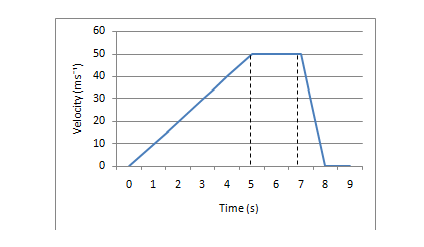Figure 2.1.5 – Velocity -Time graph

Determine its acceleration
We know that the gradient of a velocity- Time graph gives us its acceleration. Therefore for the first 5 s the acceleration is:

50/5 =10 ms?²

When the object is at constant speed from 5s to 7s its acceleration is zero. During the last second of the objects journey the object is decelerating at:

50/1 =50 ms?²

Determine its displacement
The area under a velocity-time graph is the displacement. During the first 5 s the object has travelled:

½ x 5 x 50 = 125m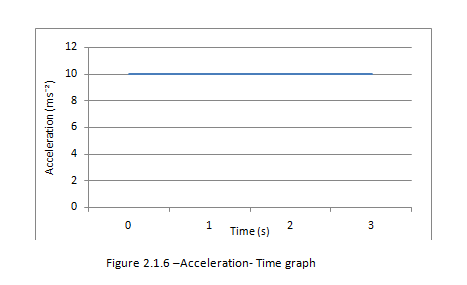Determine the change in velocity
The area under the acceleration- Time graph gives us the change in velocity

From the graph we find that the change in velocity is 10 x 3 = 30 ms?¹

Note: The gradient of the acceleration - time graph is actually the rate of change of acceleration. However it isn’t often useful.

## 2.1.9 Determine relative velocity in one and in two dimensions.

#### Problem Set 1 Solutions

```#1a:
annual_salary = float(input('Enter your annual salary: '))
portion_saved = float(input('Enter the percent of your salary to save, as a decimal:​ '))
total_cost = float(input('Enter the cost of your dream home: '))
current_savings = 0
portion_down_payment = 0.25
down_cost = total_cost * portion_down_payment
r = 0.04
monthly_salary = annual_salary/12
num_months = 0

while current_savings < down_cost:
current_savings += monthly_salary * portion_saved + current_savings * r/12
num_months += 1

num_years = num_months/12

print('Number of months:', num_months)
print('Number of years:',num_years)

#1b
annual_salary = float(input('Enter your annual salary: '))
portion_saved = float(input('Enter the percent of your salary to save, as a decimal:​ '))
total_cost = float(input('Enter the cost of your dream home: '))
semi_annual_raise = float(input('Enter the semi­annual raise, as a decimal: '))
current_savings = 0
portion_down_payment = 0.25
down_cost = total_cost * portion_down_payment
r = 0.04
monthly_salary = annual_salary/12
num_months = 0

while current_savings < down_cost:
current_savings += monthly_salary * portion_saved + current_savings * r/12
num_months += 1
if num_months%6 == 0:
monthly_salary *= (1 + semi_annual_raise)

num_years = num_months/12

print('Number of months:', num_months)
print('Number of years:',num_years)

#1c
def whatrate():
annual_salary = int(input('Enter the starting salary: '))
total_cost = 1000000
semi_annual_raise = 0.07
current_savings = 0
portion_down_payment = 0.25
down_cost = total_cost * portion_down_payment
r = 0.04
monthly_salary = annual_salary/12
num_months = 36
high = 10000
low = 0
ans = (high + low)/2
numofsteps = 0

max_savings = 0
for i in range(1,num_months+1):
max_savings += monthly_salary + current_savings * r/12
if i%6 == 0:
monthly_salary *= (1 + semi_annual_raise)
if max_savings < down_cost:
return print('It is not possible to pay the down payment in three years.')

min_savings = 0
for i in range(1,num_months+1):
min_savings += monthly_salary * 0.0001 + current_savings * r/12
if i%6 == 0:
monthly_salary *= (1 + semi_annual_raise)
if min_savings > down_cost:
return print('You don\'t really need to save, mate.')

while abs(current_savings - down_cost) > 100:
current_savings = 0
monthly_salary = annual_salary/12
numofsteps += 1
for i in range(1,num_months+1):
current_savings += monthly_salary * ans/10000 + current_savings * r/12
if i%6 == 0:
monthly_salary *= (1 + semi_annual_raise)
if current_savings > down_cost:
high = ans
else:
low = ans
ans = (high + low)/2
print('Best savings rate:', round(ans/10000,4))
print('Steps in bisection search:​', numofsteps)```

#### MIT 6.0001

# Lecture 1: What is Computation?
1.1 Topics of the courses
* iteration and recursion
* organize and modularize
* ALGORITHMS!
* complexity of algorithms
1.2 Construction of languages
* primitive constructs - numbers, strings, simple operators
* syntax - 3.2 * 5
* static semantics - syntactically valid strings have meaning; e.g. 3.2 * "hi" #static semantic error
* semantics - meaning associated with a syntactically correct string of symbols with no static semantic errors
# syntactic + static semantic + semantic = all those errors come from
1.3 Objects (or type)
1.3.1 scalar object (can not be subdivided)
* int - represents integers, ex. 5
* float - represent real numbers, ex. 3.27
* bool - represent Boolean values True and False
* Nonetype - special and has one value, None
# type() - see the type of an object
# int() or float() - type conversions (cast)
1.3.2 non-scalar (internal structure)
* list - [1, 2, 3]
1.4 Expression <object><operator><object>
# an expression has a value, which has a type
* i + j - the sum
* i - j - the difference
* i * j - the product
* i / j - the division #result is float
** i % j - the remainder when i is divided by j
** i ** j - i to the power of j
# use parentheses () to tell Python to do these operations first
1.5 Binding variables and values
* equal sign is an assignment of a value to a variable name
* pi = 3.14159; radius = 2.2; area = pi * (radius ** 2)

# Lecture 2: Branching and iteration
2.1 string object type
2.1.1 strings - letters, special characters, spaces, digits
* hi = "hello there"; name = "qixiang"; - enclose in quotation marks or single quotes
* greeting = hi + " " + name - concatenate strings
* silly = hi + " " + name * 3 - operations on string
2.1.2 INPUT/OUTPUT - print("") & input("")
* 2 way of printing sentences - print("My name is", x, "."); print("My name is " + x + " .")
2.1.3 comparision operators on int, float, string (ascii table)
* i == j - equality test
* i != j - inequality test
2.1.4 logic operators on bools
* not a - True if a is False, False if a is True
* a and b - True if both are True
* a or b - True if either or both are True
2.2 branching and conditionals (CONTROL FLOW)
2.2.1 if<condition> + <expression>
2.2.2 elif<condition> + <expression>
2.2.3 else: +<expression>
* <condition> has a value True or False
* evaluate expressions in the block if <condition> is True
2.3 indentation - how you denote (indiate) block of code
* vs == and !=
2.4 iteration and loops
2.4.1 while loops
* while <condition>: <expression>
# <condition> evaluates to a Boolean;
if <condition> is True do <expression>;
check <condition> again;
repeat until <condition> is False
* iteration through nums in a sequence - i = 0; while num < i: <expression>
2.4.2 for loops
* for <variable> in range(start, stop, step)
# loop until value is stop -1
2.4.3 break STATEMENT
* exits only innermost loop!
2.4.4 comparation between for and while LOOPS
* for LOOPS -
# known number of iterations;
with an inner counter;
rewritiable through while LOOPS
* while LOOPS -
# unbounded number of iterations;
can use a counter but must initialize;
MAYNOT rewritiable through for LOOPS

# Lecture 3: String Manipulation, Guess and Check, Approximations, Bisection
# last 3 are ALGORITHMS for guessing
3.1 string manipulation
3.1.1 definition and basic operation
* sequence of case sensitive characters
* compare operation - ==, >, < etc.
* length operation - len()
3.1.2 indexing
* square brackets to index string
# s = "abc" index: 0 1 2 or -3 -2 -1
s == s[-3] = "a"; s == s[-2] = "b"; s == s[-1] = "c"
3.1.3 slicing
* slice strings using [start: stop: step]
# s = "abcdefgh"
s[3: 6: 2] = "df"
* strings are "IMMUTABLE" - cannot be modified
# change s = "hello" to s = "yello"
s = 'y' - ERROR!
s = 'y' + s[1: len(s)]
3.1.4 LOOPS RECAP (string loops)
* loop variable
- numbers - for var in range(4, 6): <expression>
- indexing in string - for index in range(len(s)): <expression>
- CHAR - for char in s: <expression>
# more pythonic for char in word
* example - Robot cheerleader & Common letter
3.2 guess and check algorithms (exhuasted enumeration)
* for guess in range(cube + 1) - test from 0 to cube
if guess ** 3 == cube:
print("Cube root of", cube, "is", guess)
# guess a value for solution
# check if the solution is correct
# keep guessing and checking ...unitil find solution or guessed all values
3.3 approximate solutions (find an approximate solution)
* Initial value
- epsilon (how close to the target) and increment (how large each step is)
- target and guess, and num_guesses
* while abs(guess ** 3 - cube) >= epsilon and guess <=cube:
guess += increment
num_guesses += 1
# good enough approximation
# keep guessing if abs(guess ** 3 - cube) >= epsilon, for some small epsilon
# decreasing increment size - slower program
increasing epsilon - less accurate answer
3.4 bisection search method
* half interval each iteration and new guess is halfway in between
* guess CONVERGES on the order of log2N steps
* Initial low and high value - low = 0, high = guess, guess = (high + low) / 2.0
While abs(guess ** 3 - cube) >= epsilon:
if guess ** 3 < cube:
low = guess  #re-define the low boundary
else:
high = guess  #re-define the high boundary
guess = (high + low) / 2.0
num_guesses +=1

#### Introduction to Computational Thinking and Data Science

Lecture 1

1. Computational models: optimisation, simulation, statistical

2. Lambda function

3. Optimisation model: optimise with constraints

a. Brute force algorithm

b. Greedy algorithm (doesn't always yield the best result)

Lecture 2

Brute force algorithm

1. Left-most and depth-most enumeration

Paused at 13:00

#### Journal - 6 Oct 2020

It was a wonderful day.

#### Startup Lessons

Lecture 1.

• Be real builders. Real recognise real.
• Too much money, plenty of good people, and plenty of good problems to solve.

Lecture 2.

• Show, don't tell
• Don't use buzzwords
• Write using journalism's inverted pyramid

#### Introduction

Lecture 1

Concepts

• computer - calculations, storage, result memorization, - tell them what to do!
• 1. statement of facts  2. order/recipe/ the sequence of steps
• flow of control: making decisions
• 6 primitives --> types of result -- > instruction flow --> output
• expression: primitives + programming languages
• expression - value - type

[Revisit]

Programming

• 35:00
• give names, reuse names
• = assignment (left - variable)

Remaining Question:

#### `u``sa_gold = 46``uk_gold = 27``romania_gold = 1``total_gold = usa_gold + uk_gold + romania_gold``print(total_gold)``romania_gold += 1`

```    total_gold = usa_gold + uk_gold + romania_gold
print(total_gold)```

My question:

Why do we get 75 for the second print?

From John's notes:

so, romania_gold + = 1 is equal to romania_gold = romania gold + 1

#### Personality and its Transformations

Lecture 1

1. Spans a wide range of disciplines

2. We've been shaping how all of us behave as long as we've been social

3. Mythological representation and shamanic transformation

4. Piaget

5. Jung

6. Freud

Lecture 2

1. Depth psychologist

2. Constructivist

3. Humanist

4. Existentialist

5. Phenomenologist

Lecture 3

1. Archetypal stories: we somehow know what makes a story interesting

2. Constituent elements of stories: characters

#### Introduction to Psychology

Lecture 1:
With Mind Palace, memorizing a whole book can be both fun and easy.
Mind Palace is a memory technique invented by Greek poet, Simonides of Ceos, beloved by Sherlock Holmes, and used by memory champions.
Learn by doing real projects provided big companies, startups, and NGOs.
If you don't practise, you're not really learning.
If you don't know how to practise, you won't pick up a skill.
Start a company Get a job at Tesla

This feature is still in beta. Leave your email to
get early access.

Launch your career while you're learning.
With AstraConnect, you can connect with companies and organisations early and learn on-demand skills.
Or, you can connect with startup founders and investors to launch your startup--that's how Astrasum was born!

#### Friday 27 Nov 2020

Learn computer science on Astrasum ...

### Routines:

- Learn computer science on Astrasum
- Every Friday
...
Mastery comes from the power of repetition
Set your routines on AstraPanel and your daily to-dos will be automatically generated every morning.

## Acquire an in-demand skillset by doing a project

### Learn by doing. Do by learning.

×

Welcome to Astrasum

In most schools around the world, education means putting information into our brains and process them so that we can ace exams.

Nevertheless, as much as it does make us know more, most schools or educational systems often fail to make us more capable.

That is, the learning that takes place in school is mostly incomplete and does not equip us with skills that will enable us to create values that push humanity forward.

How so?

Well, if you dissect our learnin behaviour, you'll see it can be roughly divided into five steps.

1. Find learning materials
2. Input information (reading, listening, touching, smelling, tasting)
3. Process input and generate knowledge (comprehending, memorising, associating...)
4. Practise (doing a related project, solving hypothetical problems)
5. Output value by apply the new skill

The problem is, for most subjects, schools often neglect the last two steps of learning. The kind of 'practice' we do is often just repeating the third step--processing information--over and over again in preparation for exams.

That's why there's often a gap when we just come out of schools and join the workforce. This system makes some of us really good at processing information, but makes most of us really bad at doing what our jobs need us to do.

On Astrasum, you get to fill this gap in your learning by doing projects matched with skillsets you want to pick up. You learn by doing a project, and you do a project by learning all the mini-skills required at the same time.

Now, get started and unlease your learning power within.

Step 1: Get started

Pick a project matched with a skillset.

Step 2: Do by learning

Implement your project and learn required skills at the same time.

Step 3: Learn fast and furious

Use a suite of tools on Astrasum to learn better and faster.

Step 4: Take a leap

Turn your project into a real company.

Or connect with companies and increase your career prospects.